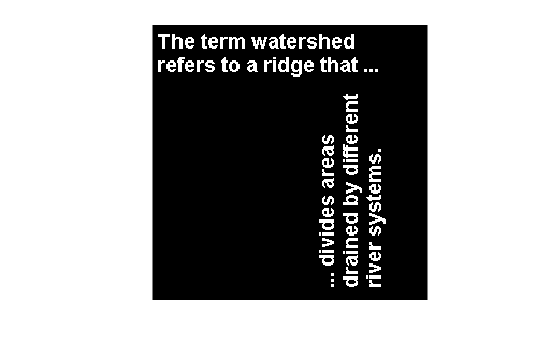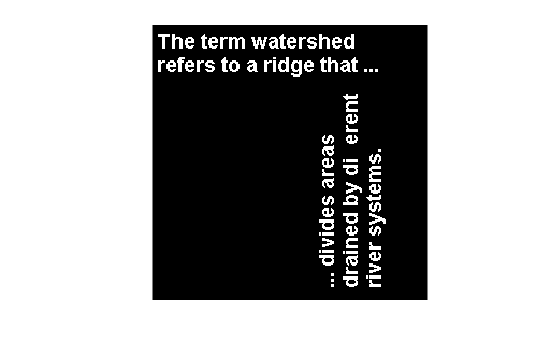# bwconncomp

Find connected components in binary image

## Syntax

``CC = bwconncomp(BW)``
``CC = bwconncomp(BW,conn)``

## Description

example

````CC = bwconncomp(BW)` returns the connected components `CC` found in the binary image `BW`. `bwconncomp` uses a default connectivity of 8 for two dimensions, 26 for three dimensions, and `conndef(ndims(BW),'maximal')` for higher dimensions.```

example

````CC = bwconncomp(BW,conn)` returns the connected components where `conn` specifies the desired connectivity for the connected components.```

## Examples

collapse all

Create a small sample 3-D array.

```BW = cat(3, [1 1 0; 0 0 0; 1 0 0],... [0 1 0; 0 0 0; 0 1 0],... [0 1 1; 0 0 0; 0 0 1]);```

Find the connected components in the array.

`CC = bwconncomp(BW)`
```CC = struct with fields: Connectivity: 26 ImageSize: [3 3 3] NumObjects: 2 PixelIdxList: {[5x1 double] [3x1 double]} ```

Calculate centroids of the objects in the array.

`S = regionprops(CC,'Centroid')`
```S=2×1 struct array with fields: Centroid ```

Read image into the workspace and display it.

```BW = imread('text.png'); imshow(BW)```Find the number of connected components in the image.

`CC = bwconncomp(BW)`
```CC = struct with fields: Connectivity: 8 ImageSize: [256 256] NumObjects: 88 PixelIdxList: {1x88 cell} ```

Determine which is the largest component in the image and erase it (set all the pixels to 0).

```numPixels = cellfun(@numel,CC.PixelIdxList); [biggest,idx] = max(numPixels); BW(CC.PixelIdxList{idx}) = 0;```

Display the image, noting that the largest component happens to be the two consecutive f's in the word different.

```figure imshow(BW)```## Input Arguments

collapse all

Input binary image, specified as a numeric or logical array of any dimension. For numeric input, any nonzero pixels are considered to be `on`.

Example: `BW = imread('text.png');`

Data Types: `single` | `double` | `int8` | `int16` | `int32` | `int64` | `uint8` | `uint16` | `uint32` | `uint64` | `logical`

Pixel connectivity, specified as one of the values in this table. The default connectivity is `8` for 2-D images, and `26` for 3-D images.

Value

Meaning

Two-Dimensional Connectivities

4-connected

Pixels are connected if their edges touch. Two adjoining pixels are part of the same object if they are both on and are connected along the horizontal or vertical direction.8-connected

Pixels are connected if their edges or corners touch. Two adjoining pixels are part of the same object if they are both on and are connected along the horizontal, vertical, or diagonal direction.Three-Dimensional Connectivities

6-connected

Pixels are connected if their faces touch. Two adjoining pixels are part of the same object if they are both on and are connected in:

• One of these directions: in, out, left, right, up, and down18-connected

Pixels are connected if their faces or edges touch. Two adjoining pixels are part of the same object if they are both on and are connected in

• One of these directions: in, out, left, right, up, and down

• A combination of two directions, such as right-down or in-up26-connected

Pixels are connected if their faces, edges, or corners touch. Two adjoining pixels are part of the same object if they are both on and are connected in

• One of these directions: in, out, left, right, up, and down

• A combination of two directions, such as right-down or in-up

• A combination of three directions, such as in-right-up or in-left-downFor higher dimensions, `bwconncomp` uses the default value `conndef(ndims(BW),'maximal')`.

Connectivity can also be defined in a more general way for any dimension by specifying a 3-by-3-by- ... -by-3 matrix of `0`s and `1`s. The `1`-valued elements define neighborhood locations relative to the center element of `conn`. Note that `conn` must be symmetric about its center element. See Specifying Custom Connectivities for more information.

Data Types: `double` | `logical`

## Output Arguments

collapse all

Connected components, returned as a structure with four fields.

FieldDescription
`Connectivity`Connectivity of the connected components (objects)
`ImageSize`Size of `BW`
`NumObjects`Number of connected components (objects) in `BW`
`PixelIdxList`1-by-`NumObjects` cell array where the k-th element in the cell array is a vector containing the linear indices of the pixels in the k-th object.

## Tips

• The functions `bwlabel`, `bwlabeln`, and `bwconncomp` all compute connected components for binary images. `bwconncomp` replaces the use of `bwlabel` and `bwlabeln`. It uses significantly less memory and is sometimes faster than the other functions.

FunctionInput DimensionOutput FormMemory UseConnectivity
`bwlabel`2-DLabel matrix with double-precisionHigh4 or 8
`bwlabeln`N-DDouble-precision label matrixHighAny
`bwconncomp`N-D`CC` structLowAny
• To extract features from a binary image using `regionprops` with default connectivity, just pass `BW` directly into `regionprops` using the command `regionprops(BW)`.

• To compute a label matrix having more memory-efficient data type (for instance, `uint8` versus `double`), use the `labelmatrix` function on the output of `bwconncomp`. See the documentation for each function for more information.

## Algorithms

The basic steps in finding the connected components are:

1. Search for the next unlabeled pixel, `p`.

2. Use a flood-fill algorithm to label all the pixels in the connected component containing `p`.

3. Repeat steps 1 and 2 until all the pixels are labeled.